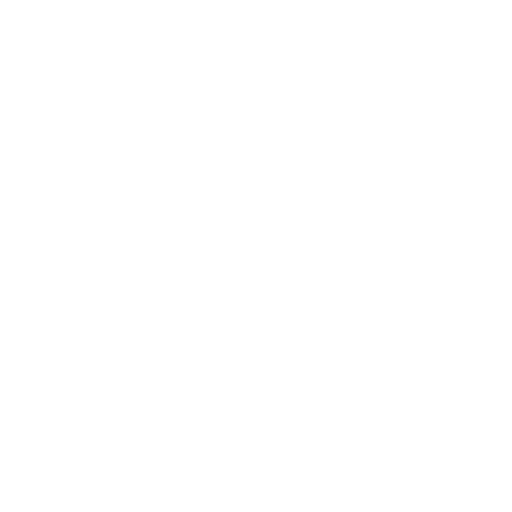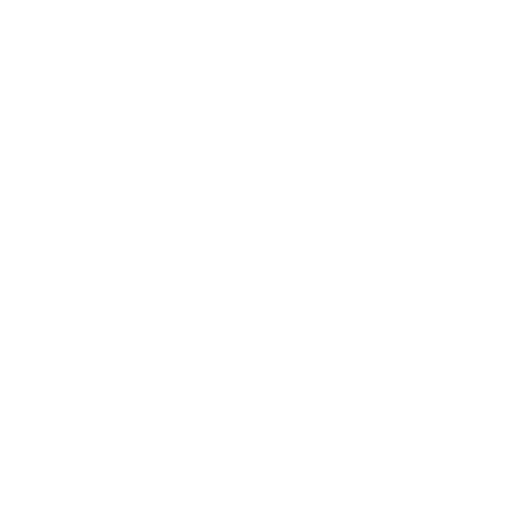Data Academy Subscription > Getting Started - Data Academy > Understanding Python's Main Tools in StatisticsIntroductory1 hour 22 minutes
Course Description
This course presents a practical introduction to the main libraries that most statistics practitioners use in Python. It will cover some of the most important and useful tools used for statistics and calculus in Python. This course is part of the Statistics and Calculus learning path – complete this path to learn how to use mathematical functions within Python.
Requirements
• As part of the Statistics and Calculus learning path, you will already have some familiarity with Python data structures and the use of functions, loops, and Jupyter notebooks.
Instructor:Packt Data